Function Repository Resource:

# ComplexRoots

Get the full list of complex nth roots of a number

Contributed by: Wolfram|AIpha Math Team
 ResourceFunction["ComplexRoots"][x,n] gives a list of the nth roots of a complex number x.

## Examples

### Basic Examples (3)

Find the three third roots of unity:

 In:=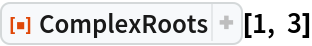Out=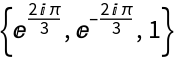Find the sixth roots of 2:

 In:=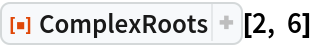Out=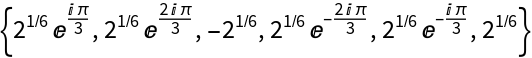Find the fifth roots of -1:

 In:=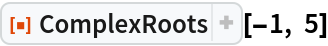Out=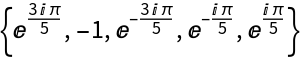### Properties and Relations (3)

Exact values are returned for exact input:

 In:=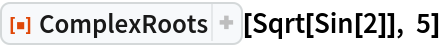Out=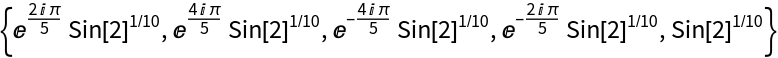Use the resource function ComplexRootQ to confirm the output of ComplexRoots:

 In:=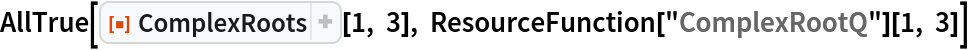Out=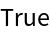The output of ComplexRoots[1,n] is similar to the output of the resource function with the exception that 1 is missing from the latter:

 In:=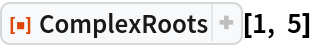Out=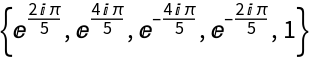In:=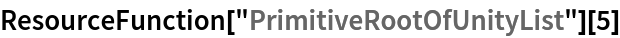Out=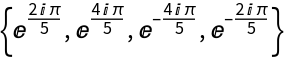## Version History

• 4.0.0 – 24 January 2020
• 3.0.0 – 06 September 2019
• 2.0.0 – 23 August 2019
• 1.0.0 – 10 July 2019